## Everything is not normal

According to the dictionary, one thing is considered normal when it’s in its natural state or conforms to standards set in advance. And this is its normal meaning. But, like many other words, “normal” has many other meanings. In statistics, we talking about normal we refer to a given probability distribution that is called the normal distribution, the famous Gauss’ bell.

This distribution is characterized by its symmetry around its mean that coincides with its median, in addition to other features which we discussed in a previous post. The great advantage of the normal distribution is that it allows us to calculate probabilities of occurrence of data from this distribution, which results in the possibility of inferring population data using a sample obtained from it.

Thus, virtually all parametric techniques of hypothesis testing need that data follow a normal distribution. One might think that this is not a big problem. If it’s called normal it’ll be because biological data do usually follow, more or less, this distribution. Big mistake, many data follow a distribution that deviates from normal. Consider, for example, the consumption of alcohol. The data will not be grouped symmetrically around a mean. In contrast, the distribution will have a positive bias (to the right): there will be a large number around zero (abstainers or very occasional drinkers) and a long right tail formed by people with higher consumption. The tail is long extended to the right with the consumption values of those people who eat breakfast with bourbon.

And how affect our statistical calculations the fact that the variable doesn’t follow a normal?. What should we do if data are not normal?.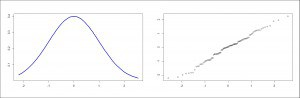The first thing to do is realize that the variable is not normally distributed. We have already seen there are a number of graphical methods that allow us to visually approximate if the data follow the normal. Histograms and box-plots allow us to test whether the distribution is skewed, if too flat or peaked, or have extreme values. But the most specific graphic for this purpose is the normal probability plot (q-q plot), where the values are set to the diagonal line if they are normally distributed.

Another possibility is to use numeric contrast tests such as the Shapiro-Wilk’s or the Kolmogorov-Smirnov’s. The problem with these tests is that they are very sensitive to the effect of sample size. If the sample is large they can be affected by minor deviations from normality. Conversely, if the sample is small, they may fail to detect large deviations from normality. But these tests also have another drawback you will understand better after a small clarification.

We know that in any hypothesis testing we set a null hypothesis that usually says the opposite of what we want to show. Thus, if the value of statistical significance is lower than a set value (usually 0.05), we reject the null hypothesis and stayed with the alternative, that says what we want to prove. The problem is that the null hypothesis is only falsifiable, it can never be said to be true. Simply, if the statistical significance is high, we cannot say it’s untrue, but that does not mean it’s true. It may happen that the study did not have enough power to reject a null hypothesis that is, in fact, false.

Well, it happens that contrasts for normality are set with a null hypothesis that the data follow a normal. Therefore, if the significance is small, we can reject the null and say that the data are not normal. But if the significance is high, we simply cannot reject it and we will say that we have no ability to say that the data are not normal, which is not the same as to say that the fit a normal distribution. For these reasons, it is always advisable to complement numerical contrasts with some graphical method to test the normality of the variable.

Once we know that the data are not normal, we must take this into account when describing them. If the distribution is highly skewed we cannot use the mean as a measure of central tendency and we must resort to other robust estimators such as the median or other parameters available for these situations.

Furthermore, the absence of normality may discourage the use of parametric contrast tests. Student’s t test and the analysis of variance (ANOVA) require that the distribution is normal. Student’s t is quite robust in this regard, so that if the sample is large (n> 80) it can be used with some confidence. But if the sample is small or very different from the normal, we cannot use parametric contrast tests.

One of the possible solutions to this problem would be to attempt a data transformation. The most frequently used in biology is the logarithmic transformation, useful to approximate to a normal distribution when the distribution is right-skewed. We mustn’t forget to undo the transformation once the contrast in question has been made.

The other possibility is to use nonparametric tests, which require no assumption about the distribution of the variable. Thus, to compare two means of unpaired data we will use the Wilcoxon’s rank sum test (also called Mann-Whitney’s U test). If data are paired we will have to use the Wilcoxon’s sign rank test. If we compare more than two means, the Kruskal-Wallis’ test will be the nonparametric equivalent of the ANOVA. Finally, remember that the nonparametric equivalent of the Pearson’s correlation coefficient is the Spearman’s correlation coefficient.

The problem is that nonparametric tests are more demanding than their parametric equivalent to obtain statistical significance, but they must be used as soon as there’s any doubt about the normality of the variable we’re contrasting.

And here we will stop for today. We could have talked about a third possibility of facing a not-normal variable, much more exotic than those mentioned. It is the use of resampling techniques such as bootstrapping, which consists of building an empirical distribution of the means of many samples drawn from our data to make inferences with the results, thus preserving the original units of the variable and avoiding the swing of data transformation techniques. But that’s another story…

## Some comparisons are not odious

It’s often said that comparisons are odious. And the truth is that it is not appropriate to compare people or things together, since each has its values and there’s no need of being slighted for doing something differently. So it’s not surprising that even the Quixote said that comparisons are always odious.

Of course, this may be said about everyday life, because in medicine we are always comparing things together, sometimes in a rather beneficial way.

Today we are going to talk about how to compare two data distributions graphically and we’ll look at an application of this type of comparison that helps us to check whether our data follow a normal distribution.

Imagine for a moment that we have a hundred serum cholesterol values from schoolchildren. What will we get if we plot the value against themselves linearly? Simple: the result would be a perfect straight line cross the diagonal of the graph.

Now think about what would happen if instead of comparing with themselves we compare them with a different distribution. If the two data distributions are very similar, the dots on the graph will be placed very close to the diagonal. If the distributions differ, the dots will go away from the diagonal, the further the more different the two distributions. Let’s look at an example.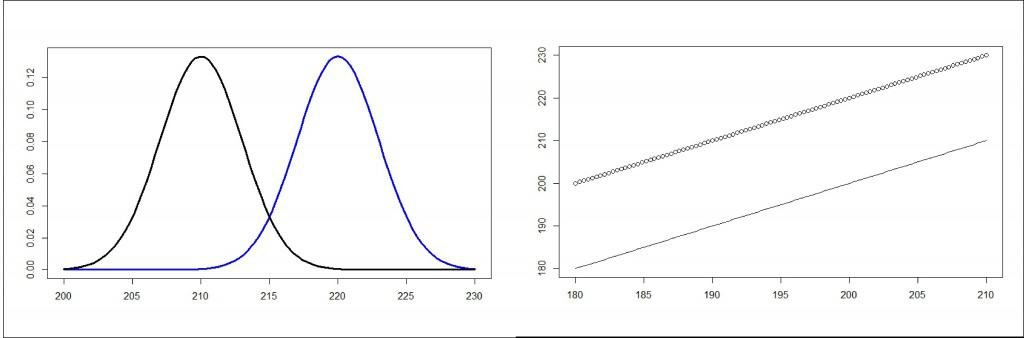Let’s suppose we divide our distribution into two parts, the cholesterol of boys and girls. According to what our imagination tells us, the boys eat more industrial bakery than the girls, so their cholesterol level are higher, as you can see if you compare the curve from girls (black) with those of children (blue). Now, if we represent the values of the girls against the values of the boys linearly, as can be seen in the figure, the dot are far from the diagonal, being evenly over it. What is the reason of this? The values of boys are higher than the values of girls.

You will tell me that all this is fine, but it can be a bit unnecessary. After all, if we want to know who have the highest values all that we have to do is look at the curves. And you will be right in your reasoning, but this type of graph has been designed for something different, which is to compare a distribution with its normal equivalent.

Imagine that we have our first global distribution and we want to know if it follows a normal distribution. We only have to calculate its mean and standard deviation and represent its quantiles against the theoretical quantiles of a normal distribution with the same mean and standard deviation. If our data are normally distributed, the dots will align with the diagonal of the graph. The more they go away from it, the less likely that our data follow a normal distribution. This type of graph is called quantile-quantile plot or, more commonly, q-q plot.

Let’s see an example of q-q plot for its better understanding. In the second graph you can see two curves, one blue colored representing a normal distribution and a black one following a Student’s t distribution. On the right side you can see the q-q plot of the Student’s distribution. Central data fits quite well the diagonal, but extreme data do it worse, varying the slope of the line. This indicates that there are more data under the tails of the distribution that the data that there would be if it were a normal distribution. Of course, this should not surprise us, since we know that the “heavy tails” are a feature of the Student’s distribution.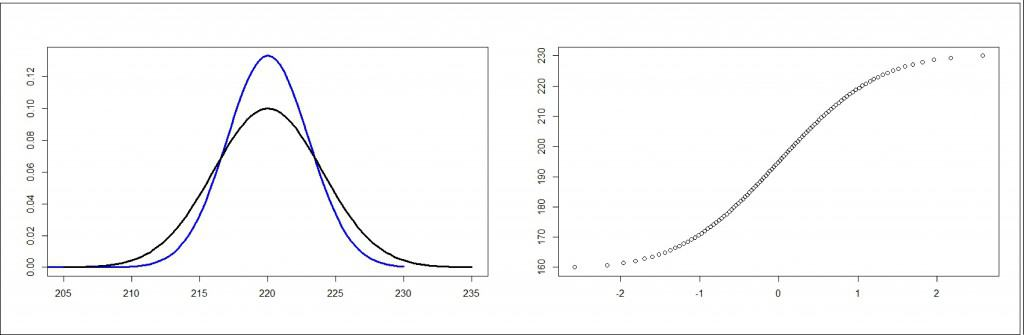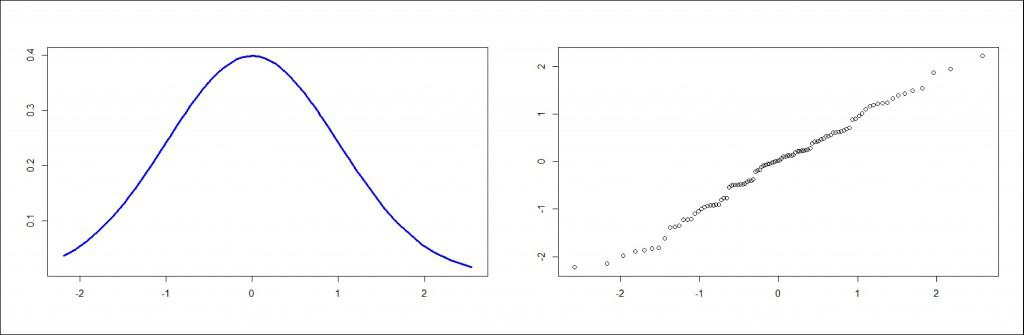Finally, in the third graph you can see a normal distribution and its q-q plot, in which we can see how the dots fit quite well to the diagonal of the graph.

As you can see, the q-q plot is a simple graphical method to determine if the data follow a normal distribution. You may say that it would be a bit tedious to calculate the quantiles of our distribution and those of the equivalent normal distribution, but remember that most statistical software can do it effortlessly. For instance, R has a function called qqnorm() that draws a q-q plot in a blink.

And here we are going to end with the normal fitting by now. Just remember that there’re other more accurate numerical methods to find out if data fit a normal distribution, such as the Kolmogorov-Smirnov’s test or the Shapiro-Wilk’s test. But that’s another story…

## The big family

Moviegoers do not be mistaken. We are not going to talk about the 1962 year movie in which little Chencho get lost in the Plaza Mayor at Christmas and it takes until summer to find him, largely thanks to the search tenacity of his grandpa. Today we’re going to talk about another large family related to probability density functions and I hope nobody ends up as lost as the poor Chencho on the film.

No doubt the queen of density functions is the normal distribution, the bell-shaped. This is a probability distribution that is characterized by its mean and standard deviation and is at the core of all the calculus of probability and statistical inference. But there’re other continuous probability functions that look something or much to the normal distribution and that are also widely used when contrasting hypothesis.

The first one we’re going to talk about is the Student’s t distribution. For those curious of science history I’ll say that the inventor of this statistic was actually William Sealy Gosset, but as he must have liked his name very little, he used to sign his writings under the pseudonym of Student. Hence the name of this statistic.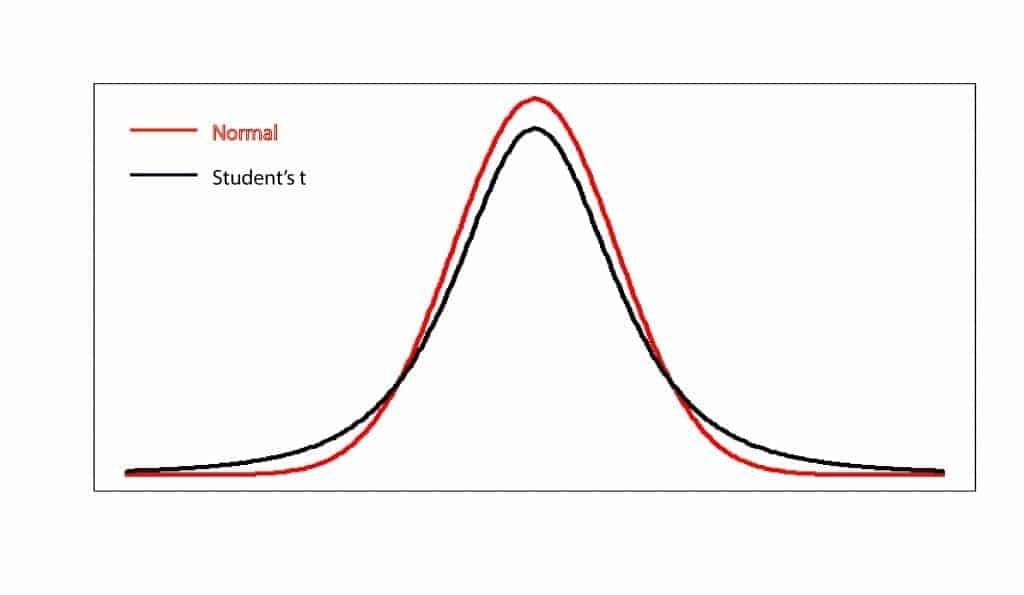This density function is a bell-shaped one that is distributed symmetrically around its mean. It’s very similar to the normal curve, although with a heavier tails; this is the reason why this distribution estimates are less accurate when the sample is small, since more data under the tails implies always the possibility of having more results far from the mean. There are an infinite number of student’s t distributions, all of them characterized by their mean, variance and degrees of freedom, but when the sample size is greater than 30 (with increasing the degrees of freedom), t distribution can be approximate to a normal distribution, so we can use the latter without making big mistakes.

Student’s t is used to compare the means of normally distributed populations when their sample sizes are small or when the values of the populations variances are unknown. And this works so because if we subtract the mean from a sample of variables and divide the result by the standard error, the value we get follows a Student’s t distribution.

Another member of this family of continuous distributions is that of the chi-square, which also plays an important role in statistics. If we have a sample of normally distributed variables and we squared them, their sum will follow a chi-square with a number of degrees of freedom equal to the sample size. In practice, when we have a series of values of a variable, we can subtract the expected values under the null hypothesis from the observed ones, square these differences, and add them up to check the probability of coming up with that value according to the density function of a chi-square. So, we will decide whether to reject or not our null hypothesis.

This technique can be used with three aims: determining the goodness of fit to a theoretical population, to test the homogeneity of two populations and to contrast the independence of two variables.

Unlike the normal distribution, chi-square’s density function only has positive values, so it is asymmetric with a long right tail. What happens is that the curve becomes gradually more symmetric as degrees of freedom increase, increasingly resembling a normal distribution.

The last distribution of which we are going to talk about is the Snedecor’s F distribution. There’s not surprise in its name about their invention, although it seems that a certain Fisher was also involved in the creation of this statistic.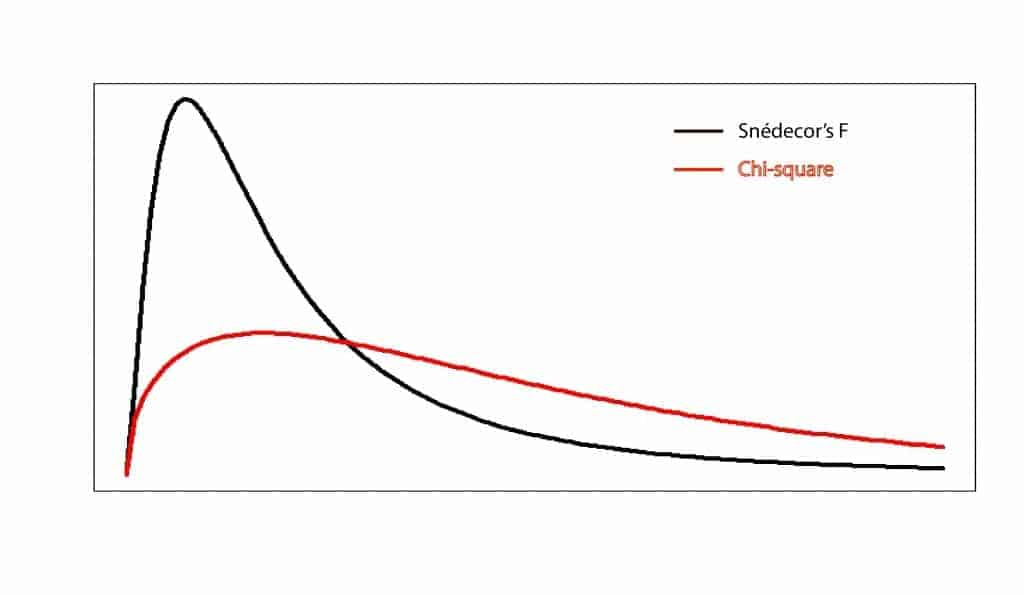This distribution is more related to the chi-square than to normal distribution, because it’s de density function of the ratio of two chi-square distributions. As is easy to understand, it only has positive values and its shape depends on the number of degrees of freedom of the two chi-square distribution that determine it. This distribution is used for the constrast of means in the analysis of variance (ANOVA).

In summary, we can see that there’re several very similar density function distributions to calculate probabilities and that are useful in various hypothesis contrast. But there’re many more, as the bivariate normal distribution, the negative binomial distribution, the uniform distribution, and the beta and gamma distributions, to name a few. But that’s another story…

## The most famous of bells

The dictionary says that a bell is a simple device that makes a sound. But a bell can be much more. I think there’s even a plant with that name and a flower with its diminutive.

But undoubtedly, the most famous of all bells is the renowned Gauss’ bell curve, the most beloved and revered by statisticians and other species of scientific.

But, what is a bell curve?. It’s nothing more, nor less, than a probability density function. Put another way, it is a continuous probability distribution with a symmetrical bell-shape, hence the first part of its name. And I say the first part because the second one is more controversial because it is not quite clear that Gauss is the father of the child.

It seems that the first who use this density function was somebody named Moivre, who was studying was happened to a binomial distribution when the sample size is large. Yet another of the many injustices of History, the name of the function is associated with Gauss, who used it some 50 years later to record data from his astronomical studies. Of course, for defense of Gauss, some people say the two of them discovered the density function independently.

To avoid controversy, we will call it from now on by its other name, different from Gauss’ bell: normal distribution. And it seems that it was so named because people used to think that most natural phenomena were consistent with this distribution. Later in time, it was found that there’re other distributions that are very common in biology, such as the binomial and Poisson’s.

As it happens with any other density function, the utility of normal curve is that it represents the probability distribution of occurrence of the random variable we are measuring. For example, if we measure the weights of a population of individuals and plot it, the graph will represent a normal distribution. Thus, the area under the curve between two given points on the x axis represents the probability of occurrence of those values. The total area under the curve is equal to one, which means that there’s a 100% chance (or a probability of one) of occurrence of any of the possible values of the distribution.

There’re infinite different normal distributions, all of them perfectly characterized by its mean and standard deviation. Thus, any point in the horizontal axis can be expressed as the mean plus or minus a number of times the standard deviation and its probability can be calculated using the formula of the density function, which I dare not so show you here. We can also use a computer to calculate the probability of a variable within a normal distribution, but what we do in practice is something simpler: to standardize.

The standard normal distribution is the one that has a mean of zero and a standard deviation of one. The advantage of the standard normal distribution is twofold. First, we know its distribution of probabilities among different points on the horizontal axis. So, between the mean plus or minus one standard deviation are 68% out of the population, between the mean and plus or minus two deviations are 95%, and between three standard deviations 99% out of the population, approximately.

The second advantage is that any normal distribution can be transform into a standard one, simply subtracting the mean to the value and dividing the result by the standard deviation of the distribution. We came up so with the z score, which is the equivalent of the value of our variable in a standard normal distribution with mean zero and a standard deviation of one.

So, you can see the usefulness of it. We do not need software to calculate the probability. We just standardize and use a simple probability table, if we do not know the value by heart. Moreover, the thing goes beyond.

Thanks to the magic of the central limit theorem, other distributions can be approximated to a normal one and be standardized to calculate the probability distribution of their variables. For example, if our variable follows a binomial distribution we can approximated it to a normal distribution when the sample size is large. In practice, when np and n(1-p) are greater than five. The same applies to the Poisson’s distribution, which can be approximated to a normal when its mean is greater than 10.

And magic is twofold because besides of being able to avoid the use of complex tools and allow us to easily calculate probabilities and confidence intervals, it should be noted that both binomial and Poisson’s distributions are discrete mass functions, while normal distribution is a continuous density function.

And that’s all for now. I only want to say that there’re other continuous density functions different from normal distribution and that they can also be approximated to a normal when the sample is large. But that’s another story…